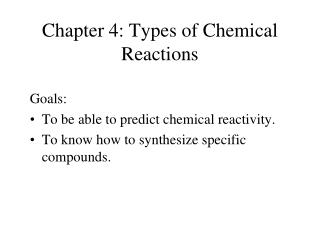DownloadDownload PresentationChapter 4: Types of Chemical Reactions

# Chapter 4: Types of Chemical Reactions

Download Presentation## Chapter 4: Types of Chemical Reactions

- - - - - - - - - - - - - - - - - - - - - - - - - - - E N D - - - - - - - - - - - - - - - - - - - - - - - - - - -
##### Presentation Transcript

1. Chapter 4: Types of Chemical Reactions Goals: • To be able to predict chemical reactivity. • To know how to synthesize specific compounds.

2. Types of Reactions • Acid-Base: proton-transfer • Oxidation-Reduction: electron-transfer • Precipitation: formation of insoluble salts • Gas Forming • Organic: • Substitution • Addition • Elimination

3. Reactions in Aqueous Solution Unless mentioned, all reactions studied this and next week occur in aqueous solution.

4. Electrolytes Strong Electrolytes: solute breaks apart to give ions in solution. NaCl  Na+ + Cl- Weak Electrolytes: solute partially breaks apart to give ions. CH3CO2H  CH3CO2- + H+happens less than 5% Nonelectrolytes: no ions formed. CH3CH2OH

5. Brønsted-Lowery Acid-Base Definitions • An acid is a substance that donates a proton (H+) to a base • A base is a substance that accepts a proton (H+) from an acid

6. Brønsted-Lowery Definitions • acid: donates a proton (H+) to a base • base: accepts a proton (H+) from an acid • Acid-base reactions can be reversible:reactantsproducts or productsreactants

7. Brønsted-Lowery Definitions • An acid is a substance that donates a proton (H+) to a base • A base is a substance that accepts a proton (H+) from an acid • Acid-base reactions can be reversible:reactantsproducts or productsreactants

8. Important Acids and Bases Strong Acids: HCl hydrochloric HBr hydrobromic HI hydroiodic HNO3 nitric H2SO4 sulfuric HClO4 perchloric Weak Acid: CH3CO2H acetic Any other acids are WEAK Strong Bases: LiOH lithium hydroxide NaOH sodium hydroxide KOH potassium hydroxide Ca(OH)2 calcium hydroxide Ba(OH)2 barium hydroxide Weak Base: NH3 ammonia

9. STRONG acids in water: 100% of acid molecules form ions: HCl(aq) + H2O(l)  H3O+(aq) + Cl-(aq) H3O+ is hydronium ion

10. WEAK acids in water, ~5% or less of acid molecules form ions (acetic, H3PO4, H2CO3)

11. Polyprotic Acidsmultiple acidic H atoms H2SO4 H+ + HSO4- HSO4-  H+ + SO42- Not all H’s are acidic: CH3CO2H

12. If H3PO4 reacts as an acid, which of the following can it not make? • 1. H4PO4+ • 2. H2PO4- • 3. HPO42- • 4. PO43-

13. If C2O42- reacts in an acid-base reaction, which of the following can it not make? • 1. H2C2O4 • 2. HC2O4- • 3. 2 CO2

14. Acid-Base Reactions Strong Acid + Strong Base HCl(aq) + NaOH(aq)  NaCl(aq) + H2O(l) acidbase “salt” water

15. Acid-Base Reactions Diprotic Acids or Bases H2SO4(aq) + NaOH(aq)  H2SO4(aq) + Ba(OH)2(aq)  HCl(aq) + Ba(OH)2(aq) 

16. Acid-Base Reactions Strong Acid + Weak Base HCl(aq) + NH3(aq)  NH4Cl(aq)

17. Acid-Base Reactions Weak Acid + Strong Base HCN(aq) + NaOH(aq)  NaCN(aq) + H2O(l) acidbase “salt” water

18. Net Ionic Equations HCl(aq) + NaOH(aq)  NaCl(aq) + H2O(l) What really happens: H+(aq) + OH-(aq)  H2O(l) Sodium ion and chloride ion are “spectator ions”

19. Reactions involving weak bases HCl(aq) + NH3(aq)  NH4+(aq) + Cl-(aq) Net-Ionic Equation: NH3(aq) + H+(aq)  NH4+(aq)

20. CH3CO2H(aq) + NaOH(aq)  • 1. CH3CO2H2+(aq) + NaO(aq) • 2. CH3CO2-(aq) + H2O(l) + Na+(aq) • 3. CH4(g) + CO2(g) + H2O(l)

21. HCN(aq) + NH3(aq)  • 1. NH4+(aq) + CN-(aq) • 2. H2CN+(aq) + NH2-(aq) • 3. C2N2(s) + 3 H2(g)

22. Solution Concentration: Molarity • Molarity = moles solute per liter of solution • 0.30 mol NH3 dissolved in 0.500 LConcentration = • Written like: [NH3] = 0.60 M

23. pH Scale • In pure water, a few molecules ionize to form H3O+ and OH–H2O + H2O  OH– + H3O+ • In acidic and basic solutions, these concentrations are not equalacidic: [H3O+] > [OH–]basic: [OH–] > [H3O+]neutral: [H3O+] = [OH–]

24. pH Scale • Measure how much H3O+ is in a solution using pH • pH < 7.0 = acidic • pH > 7.0 = basic • pH = 7.0 = neutral • Measure of H3O+ and OH–concentration (moles per liter) in a solution • As acidity increases, pH decreases

25. pH Scale • The pH scale is logarithmic:100 102 log(102) = 210 101 log(101) = 11 100 log(100) = 00.1 10–1 log(10–1) = –10.01 10–2 log(10–2) = –2 • pH = –log [H3O+] • pH if [H3O+] = 10–5? 10–9? Acidic or basic? • pH if [H3O+] = 0.000057 M?

26. Finding [H3O+] from pH [H3O+] = 10-pH What is [H3O+] if pH = 8.9?

27. pH: Quantitative Measure of Acidity • Acidity is related to concentration of H+ (or H3O+) • pH = -log[H3O+]